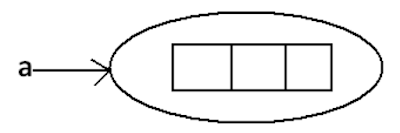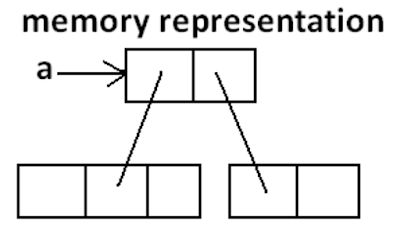## Thursday, July 21, 2022

### Language_Fundamentals : Arrays

`}`Introduction``

• An array is an indexed collection of fixed number of homogeneous data elements.
• The main advantage of arrays is we can represent multiple values with the same name so that readability of the code will be improved.
• But the main disadvantage of arrays is:
• Fixed in size that is once we created an array there is no chance of increasing or decreasing the size based on our requirement that is to use arrays concept compulsory we should know the size in advance which may not possible always.
• We can resolve this problem by using collections.

``Array declaration``

#### Single dimensional array declaration

Example:
int[] a;//recommended to use because name is clearly separated from the type
int []a;
int a[];

At the time of declaration we can't specify the size otherwise we will get compile time error.

Example:

int[] a;//valid.      int a;//invalid

#### Two-dimensional array declaration

Example:
1.     int[][] a;
2.     int [][]a;
3.     int a[][];
4.     int[] []a;
5.     int[] a[];
6.     int []a[];
All are valid.(6 ways)

Three-dimensional array declaration
Example:

1. int[][][] a;
2. int [][][]a;
3. int a[][][];
4. int[] [][]a;
5. int[] a[][];
6. int[] []a[];
7. int[][] []a;
8. int[][] a[];
9. int []a[][];
10. int [][]a[];
All are valid.(10 ways)

Which of the following declarations are valid?
1.  int[] a1,b1; //a-1,b-1 (valid)
2.  int[] a2[],b2; //a-2,b-1 (valid)
3.  int[] []a3,b3; //a-2,b-2 (valid)
4.  int[] a,[]b; //C.E: expected (invalid)
Note
• If we want to specify the dimension before the variable that rule is applicable only for the 1st variable.
• Second variable onwards we can't apply in the same declaration.

Example:

``int[] []a ,[]b;       |    |_ Invalid        |       |______ Valid ``
``Array construction``
Every array in java is an object hence we can create by using a new operator.

Example:
int[] a=new int;
Diagram:For every array type corresponding classes are available but these classes are part of java language and not available to the programmer level.

Array Type  : Corresponding class name
int[].              [I
int[][]             :  [[I
double         :   [][D

Rule 1
At the time of array creation compulsory we should specify the size otherwise we will get compile time error.

Example:
int[] a=new int;
int[] a=new int[];//C.E:array dimension missing

Rule 2
It is legal to have an array with size zero in java.
Example:
int[] a=new int; System.out.println(a.length);//0

Rule 3
If we are taking array size with -ve int value then we will get runtime exception saying NegativeArraySizeException.

Example:
int[] a=new int[-3];//R.E:NegativeArraySizeException

Rule 4
The allowed data types to specify array size are byte, short, char, int.
By mistake if we are using any other type we will get compile time error.

Example:
int[] a=new
byte b=10;
int[] a=new
short s=20;
int[] a=new
int[] a=new
int[] a=new
int['a'];//(valid)
int[b];//(valid)
int[s];//(valid)
int[10l];//C.E:possible loss of precision//(invalid)
int[10.5];//C.E:possible loss of  precision//(invalid)

Rule 5
The maximum allowed array size in java is maximum value of int size .

Example:
int[] a1=new int;(valid)
int[] a2=new int; //C.E:integer number too large:
In the first case we may get RE : OutOfMemoryError.

Multi dimensional array creation
In java multidimensional arrays are implemented as array of arrays approach but not matrix form.

The main advantage of this approach is to improve memory utilization.
Example 1
int[][] a=new int[];
a=new int;
a=new int;

Diagram:Example 2:
int[][][] a=new int[][];
a=new int[];
a=new int;
a=new int;
a=new int;
a=new int;

Diagram:

Which of the following declarations are valid?

1. int[] a=new int[]//C.E: array dimension missing(invalid)
2. int[][] a=new int;(valid)
3. int[][] a=new int[];(valid)
4. int[][] a=new int[];//C.E:']' expected(invalid)
5. int[][][] a=new int;(valid)
6. int[][][] a=new int[];(valid)
7. int[][][] a=new int[];//C.E:']' expected(invalid)

``Array Initialization``
Whenever we are creating an array every element is initialized with default value automatically.
Example 1:
int[] a=new int;
System.out.println(a);//[I@3e25a5 System.out.println(a);//0

Diagram:

Note: Whenever we are trying to print any object reference internally toString() method will be executed which is implemented by default to return the following. classname@hexadecimalstringrepresentationofhashcode.

Example 2:

System.out.println(a);//[[I@3e25a5
System.out.println(a);//[I@19821f
System.out.println(a);//0

Diagram:

Example 3:
int[][] a=new int[];
System.out.println(a);//[[I@3e25a5
System.out.println(a);//null
System.out.println(a);//R.E:NullPointerException

Diagram:

Once we created an array all its elements by default initialized with default values.
If we are not satisfied with those default values then we can replays with our customized values.

Example 4:
int[] a=new int;
a=10;
a=20;
a=30;
a=40; a=50;//R.E:ArrayIndexOutOfBoundsException: 4
a[-4]=60;//R.E:ArrayIndexOutOfBoundsException: -4

Diagram:

Note:
• if we are trying to access array element with out of range index we will get Runtime Exception saying ArrayIndexOutOfBoundsException

Declaration, construction and initialization of an array in a single line:
We can perform declaration, construction and initialization of an array in a single line.
Example:

char[] ch={'a','e','i','o','u'};(valid)
String[] s={"balayya","venki","nag","chiru"};(valid)

We can extend this short cut even for multi dimensional arrays also.
Example:
int[][] a={{10,20,30},{40,50}};

Example:
int[][][] a={{{10,20,30},{40,50}},{{60},{70,80},{90,100,110}}};

Diagram:

• int[][][] a={{{10,20,30},{40,50}},{{60},{70,80},{90,100,110}}};
• System.out.println(a);//50(valid)
• System.out.println(a);//R.E:ArrayIndexOutOfBoundsException: 2(invalid)
• System.out.println(a);//100(valid)
• System.out.println(a);//110(valid)
•  System.out.println(a);//R.E:ArrayIndexOutOfBoundsException: 2(invalid)
• System.out.println(a);//80(valid)

• If we want to use this short cut compulsory we should perform declaration, construction and initialization in a single line.
• If we are trying to divide into multiple lines then we will get compile time error.
Example:

``length Vs length():``
length:
1. It is the final variable applicable only for arrays.
2. It represents the size of the array.
Example:
int[] x=new int; System.out.println(x.length());//C.E: cannot find symbol
System.out.println(x.length);//3

length() method:
1. It is a final method applicable for String objects.
2. It returns the no of characters present in the String.
Example:
String s="bhaskar"; System.out.println(s.length);//C.E:cannot find symbol System.out.println(s.length());//7

In multidimensional arrays length variable represents only base size but not total size.
Example:
int[][] a=new int; System.out.println(a.length);//6
System.out.println(a.length);//3

Diagram:

length variable applicable only for arrays where as length()method is applicable for String objects.

There is no direct way to find total size of multi dimentional array but indirectly we can find as follows
x[o].length +x.length + x.length + .......

``Anonymous Arrays``
• Sometimes we can create an array without name such type of nameless arrays are called anonymous arrays.
• The main objective of anonymous arrays is "just for instant use".
• We can create anonymous array as follows.
• new int[]{10,20,30,40};(valid)
• new int[][]{{10,20},{30,40}};(valid)
• At the time of anonymous array creation we can't specify the size otherwise we will get compile time error.

Example:
new int{10,20,30,40};//C.E:';' expected(invalid)
new int[]{10,20,30,40};(valid)

Based on our programming requirement we can give the name for anonymous array then it is no longer anonymous.
Example:
int[] a=new int[]{10,20,30,40};(valid)

Example:
``````class Test {
public static void main(String[] args) {
System.out.println(sum(new int[]{10,20,30,40}));//100 }
public static int sum(int[] x) {
int total=0;
for(int x1:x){
total=total+x1;
}
}
} ``````
In the above program just to call sum() , we required an array but after completing sum() call we are not using that array any more, ananimous array is best suitable.

``Array element assignments``
Case 1:
In the case of primitive array as array element any type is allowed which can be promoted to declared type.

Example 1:
For the int type arrays the allowed array element types are byte, short, char, int.
int[] a=new int;
a=97;//(valid)
a='a';//(valid)
byte b=10;
a=b;//(valid)
short s=20;
a=s;//(valid)
a=10l;//C.E:possible loss of precision

Example 2:
For float type arrays the allowed element types are byte, short, char, int, long, float.

Case 2:
In the case of Object type arrays as array elements we can provide either declared type objects or its child class objects.

Example 1:
Object[] a=new Object;
a=new Integer(10);//(valid)
a=new Object();//(valid)

Example 2:
Number[] n=new Number;
n=new Integer(10);//(valid)
n=new Double(10.5);//(valid)

Diagram:

Case 3:
In the case of interface type arrays as array elements we can provide its implemented class objects.

Example:
Runnable[] r=new Runnable;

Array Type                              :  Allowed Element Type
1) Primitive arrays.                   :  Any type which can be promoted to declared type.
2) Object type arrays.                Either declared type or its child class objects allowed
3) Interface type arrays.            Its implemented class objects allowed.
4) Abstract class type arrays.    Its child class objects are allowed.

``Array variable assignments:``
Case 1:
In the case of primitive array as array element any type is allowed which can be promoted to declared type.

Example 1:
For the int type arrays the allowed array element types are byte, short, char, int.
int[] a=new int;
a=97;//(valid)
a='a';//(valid)
byte b=10;
a=b;//(valid)
short s=20;
a=s;//(valid)
a=10l;//C.E:possible loss of precision

Example 2:
For float type arrays the allowed element types are byte, short, char, int, long, float.

Case 2:
In the case of Object type arrays as array elements we can provide either declared type objects or its child class objects.

Example 1:
Number[] n=new Number;
n=new Integer(10);//(valid)
n=new Double(10.5);//(valid)

Diagram:

Case 3:
In the case of interface type arrays as array elements we can provide its implemented class objects.

Example:
Runnable[] r=new Runnable;

Array Type Allowed                  Element Type
1) Primitive arrays.                    :  Any type which can be promoted to declared type
2) Object type arrays.                :  Either declared type or its child class objects allowed
3) Interface type arrays.             : Its implemented class objects allowed.
4) Abstract class type arrays.     : Its child class objects are allowed

``Array variable assignments``
Case 1:
• Element level promotions are not applicable at array object level.
• Ex : A char value can be promoted to int type but char array cannot be promoted to int array.
Example:
int[] a={10,20,30};
char[] ch={'a','b','c'};
int[] b=a;//(valid)
int[] c=ch;//C.E:incompatible types(invalid)

Which of the following promotions are valid?

Note:
• In the case of object type arrays child type array can be assign to parent type array variable.
Example:
String[] s={"A","B"};
Object[] o=s;

Case 2:
Whenever we are assigning one array to another array internal elements won't be copy just reference variables will be reassigned hence sizes are not important but types must be matched.

Example:
int[] a={10,20,30,40,50,60,70}; int[] b={80,90};
a=b;//(valid)
b=a;//(valid)

Diagram:

Case 3:
Whenever we are assigning one array to another array dimensions must be matched that is in the place of one dimensional array we should provide the same type only otherwise we will get compile time error.

Example:
int[][] a=new int[];
a=new int;//C.E:incompatible types(invalid)
a=10;//C.E:incompatible types(invalid)
a=new int;//(valid)

Note
• Whenever we are performing array assignments the types and dimensions must be matched but sizes are not important.
Example 1:
int[][] a=new int;
a=new int;
a=new int;
a=new int;

Diagram:

Total how many objects created?
Ans: 11

How many objects eligible for GC:

Example 2 :

``````class Test {
public static void main(String[] args) {
String[] argh={"A","B"}; args=argh;
System.out.println(args.length);//2
for(int i=0;i<=args.length;i++) {
System.out.println(args[i]);
}
}
}``````

Output:

java Test x y
R.E: ArrayIndexOutOfBoundsException: 2 java Test x
R.E: ArrayIndexOutOfBoundsException: 2 java Test
R.E: ArrayIndexOutOfBoundsException: 2
Note: Replace with i<args.length

Example 3:

``````class Test {
public static void main(String[] args) {
String[] argh={"A","B"};
args=argh;
System.out.println(args.length);//2
for(int i=0;i<args.length;i++){
System.out.println(args[i]);
}
}
}``````
Output:
2
A
B

Example 4:
``````class Test {
System.out.println(args[i]);
public static void main(String[] args) {
String[] argh={"A","B"}; args=argh;
for(String s : args) {
System.out.println(s);
}
}
}``````
Output:
A
B

## You may also like

a
Kubernetes AWS Java Coding Question
Microservices Core Java Python
Spring Framework AI/MLSpring Boot